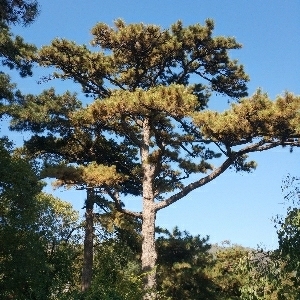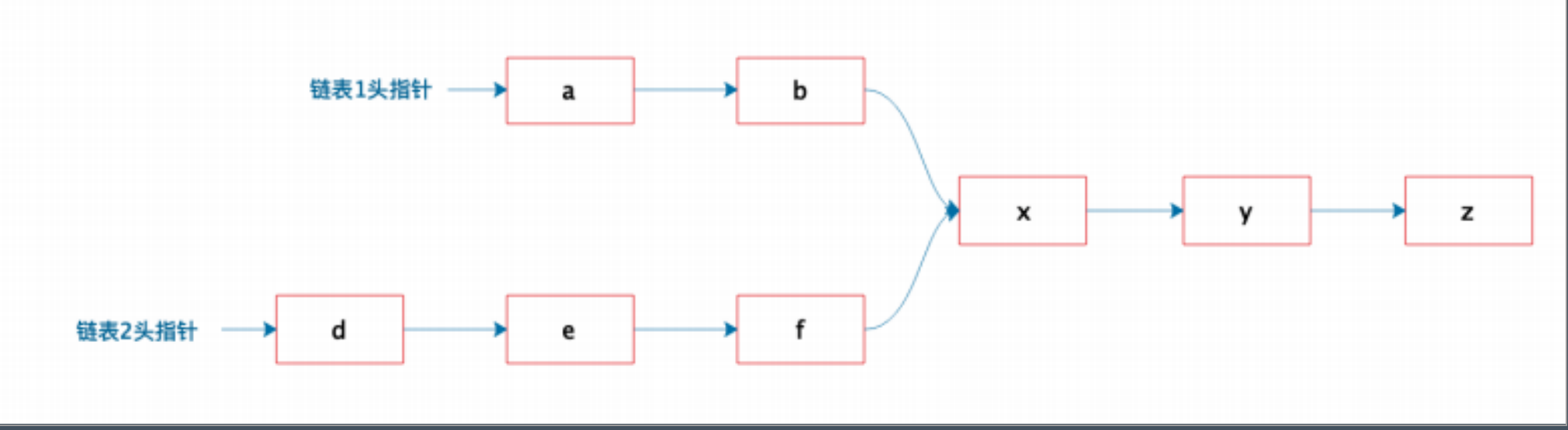# 第八周 - 习题## 习题内容

﻿## 代码实现

class Node{    public $value = null; public$nextNode = null;}class ListManager{    public $listoneFirstNode; public$listTwoFirstNode;        private function addNode(&$nodeNow,$value)    {        while (true) {            if (is_null($nodeNow)) {$nodeNow = new Node();                $nodeNow->value =$value;                break;            }            $nodeNow = &$nodeNow->nextNode;        }    }    /**     * 向第一个list添加元素     * @param $value */ public function addFirtListNode($value)    {        $this->addNode($this->listoneFirstNode, $value); } /** * 向第二个list添加元素 * @param$value     */    public function addTwoListNode($value) {$this->addNode($this->listTwoFirstNode,$value);    }    /**     * 查找两个链表的交叉点     * @return |null     */    public function findOverlappingEle()    {        //遍历第一条list        $nodeNowOneList =$this->listoneFirstNode;        while (true) {            if (is_null($nodeNowOneList)) { break; } //遍历第二条list$nodeNowSecondList = $this->listTwoFirstNode; //遍历第二条list while (true) { if (is_null($nodeNowSecondList)) {                    break;                }                //如果当前值相同，则判断后续的值是否都相同                if ($nodeNowSecondList->value ==$nodeNowOneList->value) {                    //相同，则进入后续校验                    if ($this->checkIfOverlappingEle($nodeNowOneList, $nodeNowSecondList)) { return$nodeNowSecondList->value;                    }                }                //继续下一个节点                $nodeNowSecondList =$nodeNowSecondList->nextNode;            }            $nodeNowOneList =$nodeNowOneList->nextNode;        }        return null;    }    /**     * 判断当前两个节点以及其后的节点是否都相同     * @param $nodeOne * @param$nodeTwo     * @return bool     */    private function checkIfOverlappingEle($nodeOne,$nodeTwo): bool    {        while (true) {            if (is_null($nodeOne) && is_null($nodeTwo)) {                return true;            } else if ((is_null($nodeOne) && !is_null($nodeTwo))                || (is_null($nodeTwo) && !is_null($nodeOne))                || $nodeOne->value !=$nodeTwo->value) {                return false;            }            $nodeOne =$nodeOne->nextNode;            $nodeTwo =$nodeTwo->nextNode;        }    }}

## 其他解法## 评论